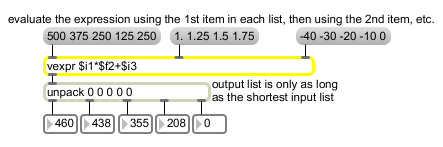# vexpr

Evaluate a math expression for a list of different inputs

## Description

The vexpr object behaves exactly like the expr object, except for the way in which it handles lists. See expr for a full description.

## Arguments

Name Type Opt Description
expression expression Obligatory. See expr.

## Messages

 bang In left inlet: Evaluates the expression and sends out the results, using the most recently received lists of numbers. int number [int] An int or float received in any inlet is treated as a single-item list. float number [float] An int or float received in any inlet is treated as a single-item list. list numbers [list] The items of each list are used individually, in order from left to right, to replace the changeable argument in a series of evaluations of the expression. When a list is received in the left inlet, the expression is first evaluated using the first item of each list, then using the second item of each list, etc. The series of results of these evaluations is then sent out as a list.

## Attributes

Name Type g/s Description
scalarmode int The word scalarmode, followed by a non-zero number, sets the scalar mode of operation. In scalar mode, sending a list of length 1 (i.e., a single value) will cause that value to be applied to each element of the other list. The message scalarmode 0 disables scalar mode.

## Output

float: If the input in one of the inlets was a single number rather than a list, and the expression is evaluated as a float value, then a single result is sent out as a float rather than a list.
int: If the input in one of the inlets was a single number rather than a list, and the expression is evaluated as an integer value, then a single result is sent out as an int rather than a list.
list: When a list is received in the left inlet, vexpr uses the first item of the lists it has received in each of its different inlets, puts those items in place of the changeable arguments in the expression, and evaluates the expression. It then does the same with the second item in each list, and so on until it has used the last item of the shortest list. It then sends out all of the different results as a single list.

## ExamplesPerform the same calculation on a whole list of input values